# Multiplying Fractions

There are lots of methods for multiplying. Some involve arrays or area models while others include algorithm.

For example, when you multiply 3 x 4, you could make a model that is 3 across and 4 down.We can use the same sort of model to multiply fractions. If you have proper fractions, you will need to start with a unit square.

Check out this example: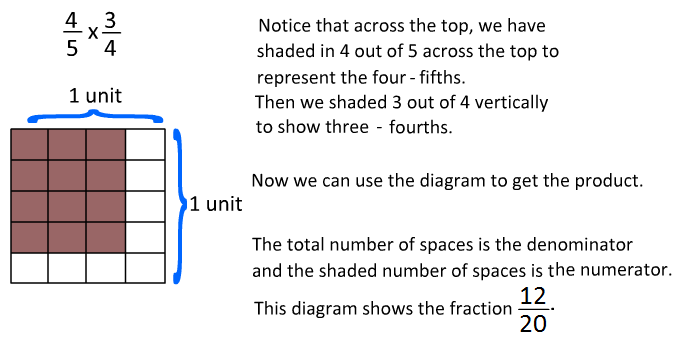So let's see what happened mathematically.When we multiply fractions, we multiply the numerators and then we multiply the denominators.

We can use this as our algorithm to multiply fractions.

Example 1: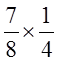Multiply the tops. Then multiply the bottoms.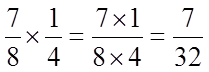Example 2:We will follow the same steps again.Example 3: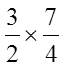In this example, we get an improper fraction. We can rewrite this as a mixed number.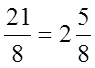Example: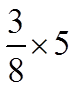We can even use this method to multiply a fraction with a whole number. The key is to write the whole number as a fraction first. 4 becomes 4 over 1. 8 becomes 8 over 1.Now we can change the improper fraction to a mixed number to show our answer.So let's review.

To multiply fractions, you simply need to multiply the numerators and then multiply the denominators. Finally, write your answer as a fraction.

Math
Fractions
Fraction Multiplication Worksheets
Math Vocabulary - Division and Fractions Quiz

Add and Subtract Like Fractions Quiz
Add and Subtract unlike fractions Quiz
Multiply Fractions Quiz
Dividing Fractions Quiz
Factors